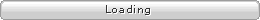﻿ OtherSide » ASP中如何按时间自动生成编号

## as level creative writing commentary

< % TempID=Year(now())&Right("00" & Month(now()),2)&Right("00" & Day(now()),2)&"001" sql="select * from table order by id desc" set rs=server.createobject("adodb.recordset") rs.open sql,conn,1,3 If Left(TempID,8)=Left(rs("number"),8) Then TempID=rs("number")+1 End If %>

exec=”insert into table(number)values(‘”&TempID&”‘)”
conn.execute exec

UPDATE@2006.10.25：感谢fisker提醒，假如数据库中还没有数据，则程序会报错，而且执行效率也有问题。现修改如下

< % TempID=Year(now())&Right("00" & Month(now()),2)&Right("00" & Day(now()),2)&"001" sql="select top 1 [number] from table order by id desc" set rs=server.createobject("adodb.recordset") rs.open sql,conn,1,3 If not rs.eof and rs.bof Then ElseIf Left(TempID,8)=Left(rs("number"),8) Then TempID=rs("number")+1 End If %>